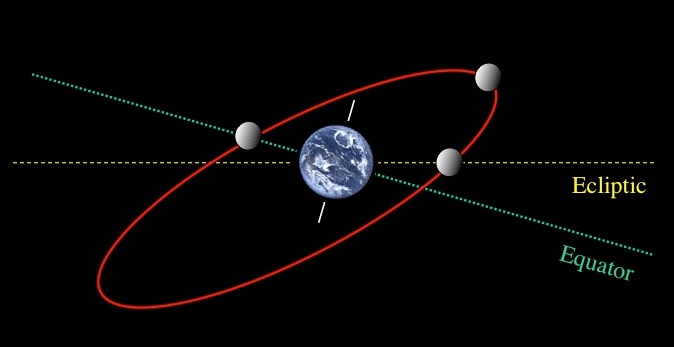# Lessons Learned from Gravity AnimationsThis entry is about programming computer animations based on the laws of physics. Gravity is probably one of the easiest and most fun computer animations to work on. Set a bunch of objects in motion and see what happens. Maybe add in a size for the objects and get some crashes, or add some walls and see these things bounce around, or…

The animations use fixed grids and fixed timesteps, similar to frames in a movie. For any number of objects, we display the objects where they are, calculate where they will get to based on their properties and display a new frame. For each object, we assign a name, a location, a mass and the difference in its location from the prior frame (the momentum).

Newton’s inverse force law says that the strength of the gravitational force between any two objects is inversely proportional to the square of their distance, i.e., the force gets smaller as objects get farther apart. More importantly, the equation tells us what the force on an object is if we know the distance to the other object.

In programming terms we have object name, mass, location x/y/z, and momentum px/py/pz. Between each frame, for each object, first calculate its distance to every other object and calculate the gravitational force on that object based on Newton’s inverse force law;  second, add the force to the momentum; finally, add the momentum to the location (px=px+forcex, x=x+px).

Click to have a look at  the Earth/Moon system and others

This is all great fun, but what did I learn.

1. Javascript using HTML5 with the built-in canvas is a great programming environment to use. It runs on all devices in all locations with no security warnings or extra downloads/installs.

2. Lots of calculations were needed that I was not expecting. If you are animating a planetary system, you need to calculate an appropriate grid size and frame rate.

These animations find the length of time for an orbit as well as the highest and lowest points of the orbit. The space station grid is laid out in meters and uses an interval of 100 seconds between frames. The Earth/Moon system uses grid spacing of 10k meters and time spacing of 20k seconds.

3. A third and important issue is the accuracy  of the starting conditions. To start the animation and get the length of time for the orbit of the earth around the sun to within an hour or two after a year, requires 4 digit accuracy in the starting location and momentum of earth. These numbers must be reverse engineered based on trial and error.

But, after getting more and more accurate through trial and error, I wondered, what about the orbit of Mercury.  Mercury has an “anomalous” precession of its perihelion. It still orbits as an ellipse, but the entire ellipse rotates around the sun. In 1915, Einstein’s general theory of relativity accounted for this “anomalous” precession and became the basis for more accurate gravity calculations.

Boy, this is exciting. I started on the orbit of Mercury and tried to make an orbit that would precess. Nothing worked despite a lot of trials. This is when I made the most amazing discovery (in my own mind of course since it is perfectly obvious).

4. No matter the starting position or momentum of the planet, every orbit ends up as an ellipse that does not rotate. There is no way to model the orbit of Mercury properly with Newton’s inverse square law.

So this presents something pretty interesting.  If we cannot use the gravitational inverse square law F=G*(M₁*M₂) / D², what equation should be used to model Mercury’s orbit correctly?

Coming soon – How to model the orbit of Mercury using relativity.